www.batmath.it

### Michelson and Morley's experiment

By supposing the existence of ether, that is the favourite frame of reference where Maxwell's equations are correct and where waves move with the speed of light, only the earth speed with respect to the ether was unknown: that velocity should not be zero, because it seemed impossible that the earth could be an absolute reference.

The earth speed with respect to the sun was already known, vTS=30 km/s. But both earth (vTE) and sun (vSE) speeds with respect to the ether were unknown: the speed that the experiment we are describing was going to measure is in effect vTE. In the following picture, to make it easier, we have considered only the ecliptic plane (in which vTS is laying for sure). As vTE=vTS+vSE, by examining this picture we can deduce that, for any sun speed with respect to the ether, there are some periods in a year where vTE≥vTS. If, in addition, vSE has a component perpendicular to the ecliptic plane, this reasoning is even more correct. There must be some periods in the year where the earth speed with respect to the ether is, at least, of 30 km/s, a non-negligible speed. The M.M.'s experience aimed to measure this speed. This experiment was done, for the first time, in 1887. There are big technical difficulties in the realization of an apparatus as the one used by M.M., but its theoretic description is conceptually simple.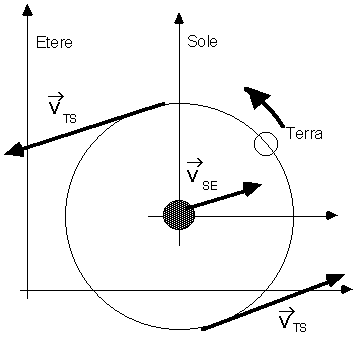S is a light source, M is a partially-silvered mirror, M1 and M2 are two mirrors, A is a shield. A beam emitted by S is splitted in two by M: the first follows the path MM1MA, the second follows the path MM2MA. Both are partially sent back to the source, but partially they reach the shield A where they will cause some interference fringes because they reach A with different times due to the path difference. It is obvious that the fringes will be determined by the different times employed by the beams to complete the MM1M and MM2M walks respectively. We will call those times t1 and t2. With calculations done (to make them easier) partially in the earth's reference system, partially in the ether's reference system, we found that the difference between the two times is given by: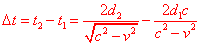.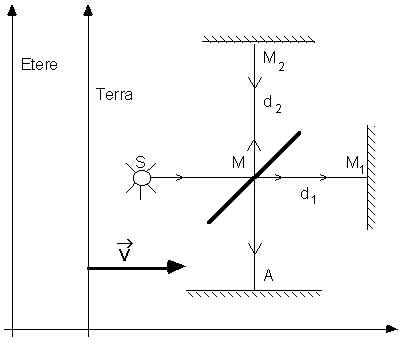If we rotate the interferometer as in the following figure the roles of t2 and t1 are reversed: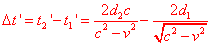, therefore a change in the interference pattern must occur, at least in certain periods of the year.

We observe explicitly  that the transformations of Galileo have been extensively used in this calculation, particularly the law of composition of speeds, applied to the speed of the light. The result of the experiment was absolutely negative: the rotation of the apparatus doesn't cause any change in the interference pattern.

It is worth to observe that the sensibility of the tool was sufficiently high, in order to reveal any change.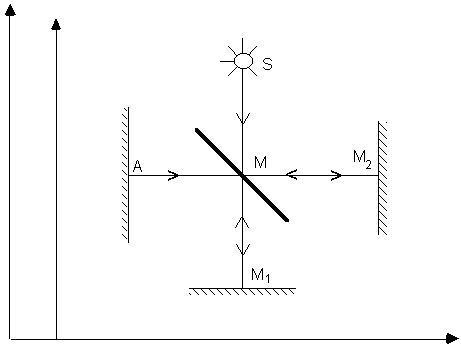;

This result, absolutely unpredictable on the bases of the known laws, gave rise to serious problems of interpretation. Some of the possibilities to explain this fact are the following:

1. The earth is fixed with respect to the ether.
2. The earth partially drives the ether.
3. Maxwell's equations are wrong. This possibility was already considered unacceptable at the end of the last century, especially thanks to the verifications of the existence of the electromagnetic waves that was done just in the years of M.M.'s experiment.
4. Light is not a wave, but it is made up of corpuscles that follow Newton's dynamic laws.
5. The result of the experiment has to be taken for what it is, and we have to revise the concepts of physics used to make the calculations that do not correspond. This was, as everybody knows, the correct solution, proposed by Einstein in 1905, which had already been suggested by some ideas, in particular those of Loretz and Poincarè. It is clear that this idea should have lead, as it actually happened, to the revision of the Newtonian mechanics and its laws: the Newtonian mechanics had to be totally revised to adapt itself to a newborn theory despite its careful explanation of the motion of  heavenly bodies.

As far as this matter is concerned we must notice that Einstein's idea perfectly agrees with the experimental method adopted, after Galileo's work, as a basis of scientific research.

first published on march 11 2002 - last updated on september 01 2003